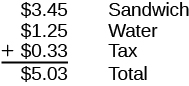Learning Outcomes

• Subtract decimals that require borrowing

Let’s take one more look at the lunch order from the start of Decimals, this time noticing how the numbers were added together.All three items (sandwich, water, tax) were priced in dollars and cents, so we lined up the dollars under the dollars and the cents under the cents, with the decimal points lined up between them. Then we just added each column, as if we were adding whole numbers. By lining up decimals this way, we can add or subtract the corresponding place values just as we did with whole numbers.

1. Write the numbers vertically so the decimal points line up.
2. Use zeros as place holders, as needed.
3. Add or subtract the numbers as if they were whole numbers. Then place the decimal in the answer under the decimal points in the given numbers.

example

Add: $3.7+12.4$

Solution

 $3.7+12.4$ Write the numbers vertically so the decimal points line up. $\begin{array}{c}\hfill 3.7\\ \hfill \underset{\text{_____}}{+12.4}\end{array}$ Place holders are not needed since both numbers have the same number of decimal places. Add the numbers as if they were whole numbers. Then place the decimal in the answer under the decimal points in the given numbers. $\begin{array}{c}\hfill \stackrel{1}{3}.7\\ \hfill \underset{\text{_____}}{+12.4}\\ \hfill 16.1\end{array}$

example

Add: $23.5+41.38$

try it

In the following video we show another example of how to add decimals.

Subtracting decimals

example

How much change would you get if you handed the cashier a $20$ bill for a $14.65$ purchase? We will show the steps to calculate this in the next example.

Subtract: $20 - 14.65$

example

Subtract: $2.51 - 7.4$

try it

This video shows another example of how to subtract one decimal from another.